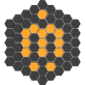# Move Object along vector

Taking the script I have below. How can I move the small green helper to be 10 units off of the light blue helper? But in the direction of the vector created from the Blue - Lightblue helpers?

```(
delete objects

clearlistener()
/* Create Corner Points */
startPt = point pos:([random -10 10,random -10 10,random 0 10]) wirecolor:blue size:4
endPt = point pos:([random -30 30,random -30 30,random 0 30]) wirecolor:[0,255,255] size:4

nvec = (normalize startPt.pos)
d = (distance endPt.pos startPt.pos)
point pos:(nvec*(d)) size:2 wirecolor:green
)```

## Comment viewing options### Easy:

```(
delete objects

clearlistener()
/* Create Corner Points */
startPt = point pos:([random -10 10,random -10 10,random 0 10]) wirecolor:blue size:4
endPt = point pos:([random -30 30,random -30 30,random 0 30]) wirecolor:[0,255,255] size:4

dir = normalize (startPt.pos - endPt.pos)
point pos:(endPt.pos + (dir * 10)) size:2 wirecolor:green
)```

Optimize the script if you want. :)